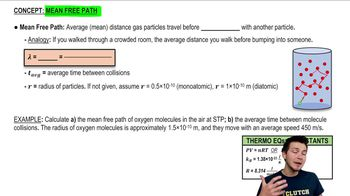Start typing, then use the up and down arrows to select an option from the list.
2:57 minutes
Problem 18
Textbook Question

# Calculate the mean free path of air molecules at 3.50 * 10^-13 atm and 300 K. (This pressure is readily attainable in the laboratory; see Exercise 18.23.) As in Example 18.8, model the air molecules as spheres of radius 2.0 * 10^-10 m.Verified Solution
This video solution was recommended by our tutors as helpful for the problem above.
62views